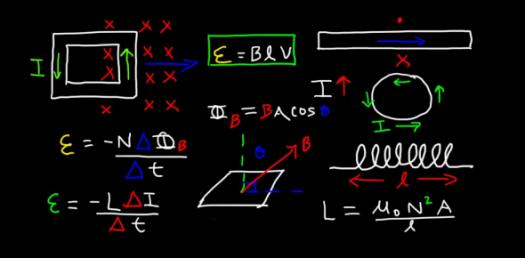# Physics Quiz: Electromagnetic Induction Trivia Questions!

14 Questions | Total Attempts: 2466Settings• 1.
Electromagnetic induction is not used in
• A.

Room heater

• B.

AC generator

• C.

Choke coil

• D.

Transformer

• 2.
A coil of area of cross section with 10 turns is in a plane which is pendendicular to an uniform magnetic field of The flux though the coil is
• A.

1 Wb

• B.

10 Wb

• C.

100 Wb

• D.

Zero

• 3.
Lenz’s law is in accordance with the law of
• A.

Conservation of energy

• B.

Conservation of charges

• C.

Conservation of momentum

• D.

conservation of flux

• 4.
The self−inductance of a straight conductor is
• A.

Zero

• B.

Infinity

• C.

Very large

• D.

Very small

• 5.
The unit henry can also be written as
• A.

(4)

• B.

(2)

• C.

(3)

• D.

(1)

• 6.
An emf of 12 V is induced when the current in the coil changes at the rate of . The coefficient of self induction of the coil is
• A.

0.3 H

• B.

0.003 H

• C.

30 H

• D.

4.8 H

• 7.
A DC of 5A produces the same heating effect as an AC of
• A.

5A rms current

• B.

5 A peak current

• C.

50 A rms current

• D.

None of these

• 8.
Transformer works on
• A.

AC only

• B.

DC only

• C.

Both AC and DC

• D.

AC more effectively than DC

• 9.
The part of the AC generator that passes the current from the coil to the external circuit is
• A.

Brushes

• B.

Split rings

• C.

Field magnet

• D.

Slip rings

• 10.
In an AC circuit the applied emf e = Eo sin (ωt + π/2) leads the current I = Io sin (ωt – π/2) by
• A.

π

• B.

π/4

• C.

π/2

• D.

0

• 11.
Which of the following cannot be stepped up in a transformer?
• A.

Input power

• B.

input current

• C.

Input voltage

• D.

All

• 12.
The power loss is less in transmission lines when
• A.

Voltage is more but current is less

• B.

Voltage is less but current is more

• C.

Both voltage and current are more

• D.

Both voltage and current are less

• 13.
Which of the following devices does not allow d.c. to pass through?
• A.

Capacitor

• B.

Resistor

• C.

inductor

• D.

All the above

• 14.
In an ac circuit
• A.

The average value of current is zero

• B.

the average value of square of current is zero.

• C.

The average power dissipation is zero.

• D.

The rms current is 2 time of peak current.

Related TopicsBack to top